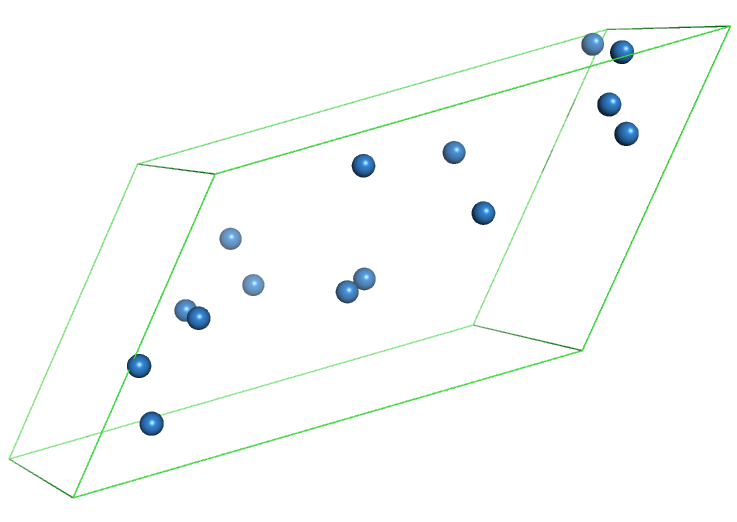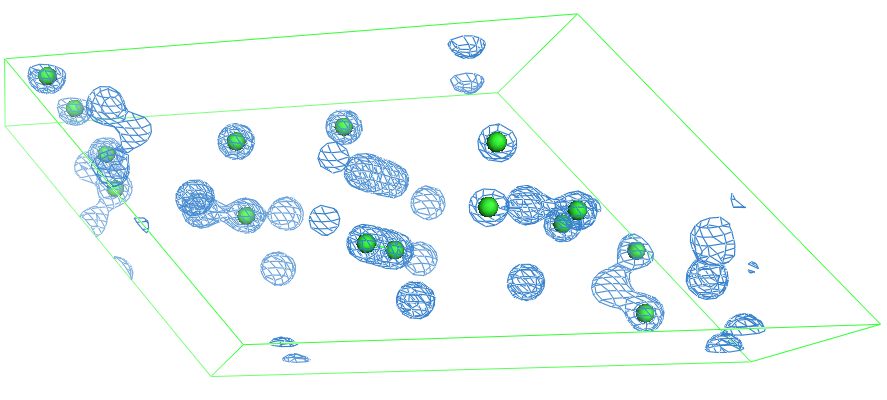## Create a random model and calculate its density

This example script shows how to generate a small centrosymmetric unit cell in P-1 with 15 atoms, and how to calculate phases and amplitudes to reconstruct the electron density.

### Required imports

This script requires the following imports:

from __future__ import absolute_import, division, print_function
import iotbx.pdb
import iotbx.mrcfile
import mmtbx.model
import mmtbx.real_space
from scitbx.array_family import flex
from cctbx.development import random_structure
from cctbx import sgtbx
from cctbx import maptbx


### Create a random structure and save as PDB

Use the random_structure() method from cctbx.development to generate a random structure that has:

• spcae group P-1
• unit cell dimensions a=10, b=20, c=30, alpha=70, beta=80, gamma=120
• 15 carbon atoms
# Create random structure
xrs = random_structure.xray_structure(
space_group_info = sgtbx.space_group_info("P-1"),
elements         = ["C"]*15,
unit_cell        = (10, 20, 30, 50, 60, 80))


Create a model object from this structure:

# Create model object
model = mmtbx.model.manager.from_sites_cart(
sites_cart       = xrs.sites_cart(),
crystal_symmetry = xrs.crystal_symmetry(),
resname          = 'DUM')


from_sites_cart() is a convenience function to create a model object from a list of cartesian coordinates. The default is to use atom name CA, scatterer C, occ 1, b_iso 30, resname GLY, residue numbers starting with 1. Here, the residue name is set to 'DUM', which stands for dummy residue. Note that even if we specify different elements in random_structure(), we will get CA atoms, unless the from_sites_cart() method is supplied with a list of atom names and scatterer names.

Save the model as PDB file:

# Write it into PDB file
with open("model.pdb","w") as fo:
fo.write(model.model_as_pdb())


Here is an example for a model that was created using this procedure (your model will look different, as the procedue will place the atoms at random places):### Create a map from a PDB file

Create a model object from a file.

  # Read the model file
pdb_inp = iotbx.pdb.input(file_name = "model.pdb")
model = mmtbx.model.manager(model_input = pdb_inp)
xrs = model.get_xray_structure()


Calculate structure factors from the model using a high resolution limit of 2 Angstrom. This will give a Fourier map:

# Calculate structure factors at given resolution.
f_calc = xrs.structure_factors(d_min = 2.0).f_calc()


For book-keeping: save the Fourier map as MTZ file:

# Write them down as MTZ file
mtz_dataset = f_calc.as_mtz_dataset(column_root_label="F-calc")
mtz_object = mtz_dataset.mtz_object()
mtz_object.write(file_name = "f_calc.mtz")


Perform a fast-Fourier-transform (FFT) to convert the structure factors into a real map:

# Convert Fcalc into real map (just do FFT)
fft_map = f_calc.fft_map(resolution_factor=1./4)
fft_map.apply_sigma_scaling()


Write the map data into an MRC file:

# Write real Fourier map into MRC file
iotbx.mrcfile.write_ccp4_map(
file_name   = "fourier_map.mrc",
unit_cell   = f_calc.unit_cell(),
space_group = f_calc.crystal_symmetry().space_group(),
map_data    = map_data.as_double(),
labels      = flex.std_string(["Some text"]))


The figure below shows the density overlayed to the model. Note that there are density peaks that are (seemingly) without model. This is because the density was calculated for the unit cell, which is P-1 (centrosymmetric). The spheres only represent the atoms in the asymmetric unit.Another option is to calculate the exact map. This requires first the computation of the grid (crystal_gridding).

# Calculate exact map and write it down
crystal_gridding = maptbx.crystal_gridding(
unit_cell        = xrs.unit_cell(),
space_group_info = xrs.space_group_info(),
symmetry_flags   = maptbx.use_space_group_symmetry,
step             = 0.3)
m = mmtbx.real_space.sampled_model_density(
xray_structure = xrs,
n_real         = crystal_gridding.n_real())
map_data = m.data()


Save the exact map in the file exact_map.mrc

iotbx.mrcfile.write_ccp4_map(
file_name   = "exact_map.mrc",
unit_cell   = f_calc.unit_cell(),
space_group = f_calc.crystal_symmetry().space_group(),
map_data    = map_data.as_double(),
labels      = flex.std_string(["Some text"]))